# How to use REGEXEXTRACT Function in Google Sheets?(With Examples)

REGEXEXTRACT function in google sheets extracts the first matching substrings according to a regular expression. Regular expressions are sophisticated search patterns or character sequences that allow you to find specific patterns in a string. Once found, they can be replaced with any other text using the REGEXEXTRACT function.

The REGEXEXTRACT is one of the three regex functions in google sheets along with the REGEXREPLACE and REGEXMATCH functions.

## What Does the REGEXEXTRACT Function in Google Sheets Do?

The REGEXEXTRACT function in google sheets extracts the first matching substrings according to a regular expression. The REGEX function is called a regular function that matches a particular pattern and replaces it with different text.

## Syntax of the REGEXEXTRACT Function in Google Sheets

=REGEXEXTRACT(text, reg_exp)

In the above syntax,

• text – is the text or string that has to be replaced
• reg_exp – is the regular expression with a particular pattern. This expression matches part of the text or string that has to be replaced. The regular expression parameter should be provided in double-quotes

## Application of the REGEXEXTRACT Function in Google Sheets

When you want to extract a set of strings that are not exactly similar or consistent in format, the REGEXEXTRACT function can be quite useful.

Below are some of the useful applications of the REGEXEXTRACT function in google sheets,

• It can be used to extract the first or last few characters from a string
• It can be used to extract numbers from a string
• It can be used to extract whole words based on a partial match
• It can be used to extract one of a list of words
• It can be used to extract contents between certain characters
• It can be used to extract different parts of a URL
• It can be used to extract different parts of email addresses

There are many other ways also in which you can use the REGEXEXTRACT function in Google sheets.

Let’s see in detail how the REGEXEXTRACT function is used in the above application below,

### Using the REGEXEXTRACT Function in Google Sheets to Extract the First or Last few Characters from a String

Here let’s see how to extract the first or last few characters in a string using the REGEXEXTRACT function in google sheets from the selected cell,

Extract the First few Characters from a String:

To extract the first few characters from a string we can use the REGEXEXTRACT function in the google sheets.

The below formula can be used to extract the first few characters,

=REGEXEXTRACT(text,”…”)

In the above expression, the three dots represent “…” the first three characters. If you need to extract only the first two characters then you could give two dots in double quotes “..”

Example,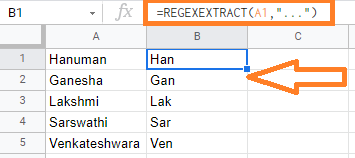In the above example, we are extracting the first three characters from the string with the expression, =REGEXEXTRACT(A1, “…”)

Extract the Last few Characters from a String:

To extract the last few characters from a string we can use the REGEXEXTRACT function in the google sheets.

The below formula can be used to extract the last few characters,

=REGEXEXTRACT(text,”…\$”)

In the above expression, the dollar(\$) symbol is suffixed to the dots. above expression represent “…\$” the last three characters. If you need to extract only the last two characters then you could give two dots ending with dollar symbol in double quotes “..\$”

Example,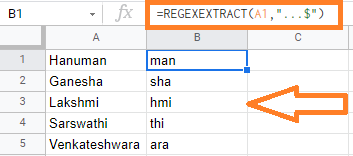In the above example, we are extracting the last three characters from the string with the expression, =REGEXEXTRACT(A1, “…\$”)

Extract the first word from a String:

Additionally, to extract only alphanumeric characters, then you need to use \w metacharacter which represents a single alphanumeric character (a digit, a letter, or an underscore) instead of the dot symbols.

Suppose if you have the first name and last name and you need to extract the first name alone, then you could use below expression,

=REGEXEXTRACT(A1,”\w+”)

In the above expression, “\w+” is used to extract the first word in the given text.

Example,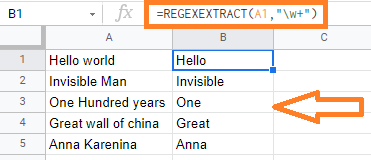In the above example, we are extracting the first word with the expression, =REGEXEXTRACT(A1, “\w+”)

Extract the last word from a String:

To extract the last name or the word then you could use the below expression,

=REGEXEXTRACT(A1, “\w+\$”)

In the above expression, “\w+\$” is used to extract the last word in the given text. We are suffixing with the dollar symbol (\$)

Example,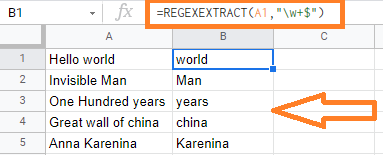In the above example, we are extracting the last word with the expression, =REGEXEXTRACT(A1, “\w+\$”)

### Using the REGEXEXTRACT Function in Google Sheets to Extract Numbers from a String

Here let’s see how to extract the numbers in a string using the REGEXEXTRACT function in google sheets from the selected cell.

Extract the first numbers from a string:

To extract the first numbers from the String, we can use the expression “\d+”. The \d metacharacter represents the numeric digit.

Below expression can be used to extract the first numbers in the string,

=REGEXEXTRACT(text, “\d+”)

Example,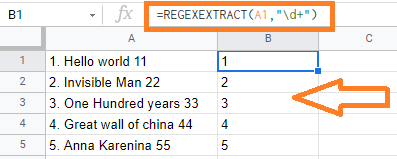In the above example, the first numbers are extracted with the expression, =REGEXEXTRACT(A1, “\d+”)

Extract the last numbers from a string:

To extract the last numbers from the String, we can use the expression “\d+\$”. The \d metacharacter represents the numeric digit. The dollar symbol (\$) is suffixed to extract the last numbers

Below expression can be used to extract the last numbers in the string,

=REGEXEXTRACT(text, “\d+\$”)

Example,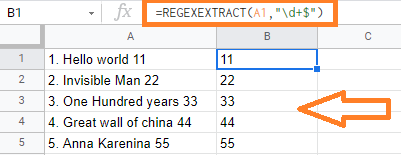In the above example, the last numbers are extracted with the expression, =REGEXEXTRACT(A1, “\d+\$”)

### Using the REGEXEXTRACT Function in Google Sheets to Extract Whole words based on Partial Match

Here let’s see how to extract whole words based on Partial Match in a string using the REGEXEXTRACT function in google sheets from the selected cell.

Example:

To extract the word which is matching the starting letter ‘bo’ and ending with the letter ‘d’, we use the below expression,

=REGEXEXTRACT(A1,”bo\w+d”)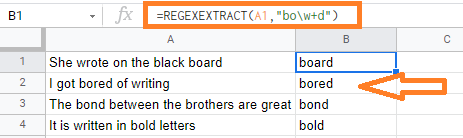In the above example, you could see that the string that staring with the letter “bo” and ending with “d” are extracted.

### Using the REGEXEXTARCT Function in Google Sheets to Extract One of a List of Words

Here let’s see how to extract one of a list of words in a string using the REGEXEXTRACT function in google sheets from the selected cell.

The or operation is represented by the metacharacter ‘|’. If you want to extract one word from a collection of words or characters, you can use the REGEXMATCH function in Google Sheets.

Example: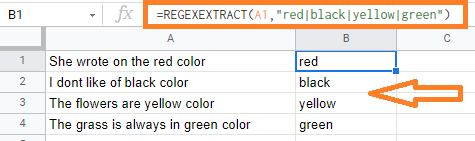In the above example, we are extracting few words i.e., red, black, yellow, and green in all the selected cells with the expression =REGEXEXTRACT(A1, “red|black|yellow|green”)

### Using the REGEXEXTRACT Function in Google Sheets to Extract Contents between Certain Characters

Here let’s see how to extract contents between certain characters using the REGEXEXTRACT function in google sheets from the selected cell.

Example: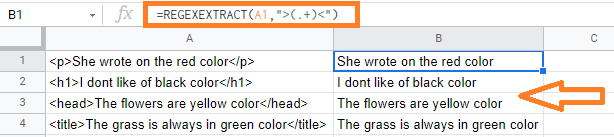In the above example, we are trying to remove the HTML tags with the expression          =REGEXEXTRACT(A1, “>(.+)<“). Here we are searching for the first symbol starting with “>” and the first symbol starting with “<“. So the text in between the above-specified symbols is returned.

### Using the REGEXEXTRACT Function in Google Sheets to Extract Different Parts of a URL

Here let’s see how to extract different parts of a URL in the string using the REGEXEXTRACT function in google sheets from the selected cell.

The below expression can be used to extract the domain name from the URL,

=REGEXEXTRACT(A1,”http.+\ / \ /(.+)\ /”)

The above expression will extract all the contents in between the pattern “HTTP://” and the “/” symbol

Example: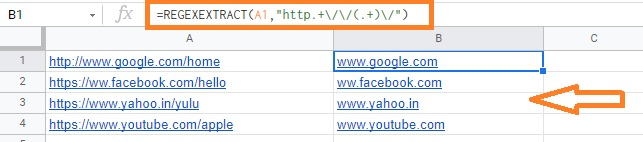In the above example, you could see we have extracted only the email domain name.

### Using the REGEXEXTRACT Function in Google Sheets to Extract Different Parts of an Email Address

Here let’s see how to extract different parts of an Email Address using the REGEXEXTRACT function in google sheets from the selected cell.

To extract only the username in the email address you can use the below expression,

=REGEXEXTRACT(A1,”(.+)@”)

Example: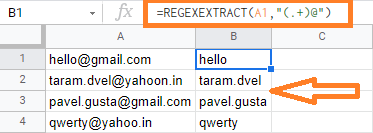In the above example, we have extracted only the username in the email address.